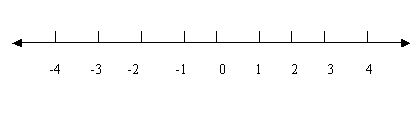Email us to get an instant 20% discount on highly effective K-12 Math & English kwizNET Programs!

#### Online Quiz (WorksheetABCD)

Questions Per Quiz = 2 4 6 8 10

### Grade 3 - Mathematics4.1 Using Number Lines

 Number Line: A number line shows the numbers in order. Numbers to the right of zero are positive numbers. Numbers to the left are negative numbers. A number line has arrows because the numbers keep going forever. You can use the number line to practice the addition and subtraction.Method: Draw a number line with numbers. To find difference, we first go forward till we reach the bigger number and then backward till we reach the smaller number. To find sum, we first go till the first number and then move further from there to reach the next number. Directions: Answer the following questions. 1) Draw a number line and show zero, positive numbers and negative numbers. 2) Also write at least ten examples with subtraction and addition using number line.
 Q 1: You can write a positive number with or without a positive sign.FalseTrue Q 2: Fill in the blank on a number line -1,0,1,2,3,4,5,6,7,8,9,___110100 Question 3: This question is available to subscribers only! Question 4: This question is available to subscribers only!

#### Subscription to kwizNET Learning System offers the following benefits:

• Unrestricted access to grade appropriate lessons, quizzes, & printable worksheets
• Instant scoring of online quizzes
• Progress tracking and award certificates to keep your student motivated
• Unlimited practice with auto-generated 'WIZ MATH' quizzes